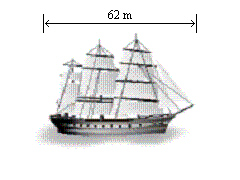Name:    Chapter 7 Test

Multiple Choice
Identify the choice that best completes the statement or answers the question.

Tell whether the angles are adjacent or vertical. Then find the value of x.

1.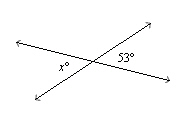a. vertical; 53 c. vertical; 127 b. adjacent; 127 d. adjacent; 53

2.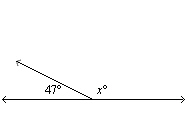a. vertical; 47 c. adjacent; 47 b. vertical; 133 d. adjacent; 133

3.

Tell whether the angles are complementary or supplementary. Then find the value of h.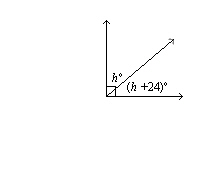a. supplementary;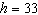c. supplementary;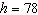b. complementary;d. complementary;4.

Tell whether the angles are complementary or supplementary. Then find the value of h.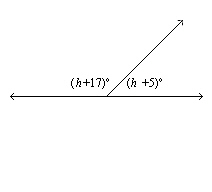a. supplementary;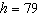c. complementary;b. complementary;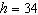d. supplementary;5.

Find the value of z. Then classify the triangle.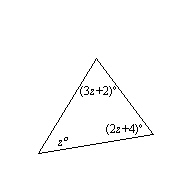a. 29; acute scalene triangle c. 29; acute isosceles triangle b. 30; right scalene triangle d. 30; obtuse scalene triangle

6.

Find the value of z. Then classify the triangle.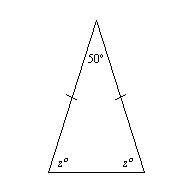a. 65; acute isosceles triangle c. 75; right scalene triangle b. 75; obtuse isosceles triangle d. 65; acute scalene triangle

7.

Find the value of z. Then classify the triangle.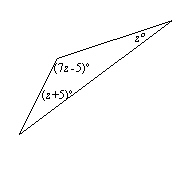a. 20; obtuse scalene triangle c. 25; acute scalene triangle b. 25; right isosceles triangle d. 20; obtuse isosceles triangle

Find the value of x.

8.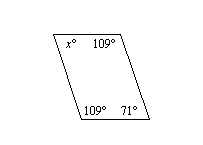a. 62 c. 65 b. 71 d. 109

9.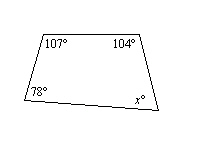a. 66 c. 65 b. 71 d. 78

10.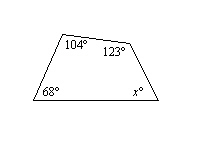a. 72 c. 60 b. 65 d. 68

Find the missing dimension. Use the scale factor 1:12.

11.

 Item Model Actual Water Tower Height: 278 cm Height: __?__ m
 a. 2316.667 m c. 3336 m b. 33.36 m d. 3.336 m

12.

 Item Model Actual Baseball Bat Length: 2.625 in. Length: __?__ in.
 a. 32.5 in. c. 31.5 in. b. 0.219 in. d. 30 in.

Draw a triangle with the given angle measures. Then classify the triangle.

1.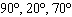2.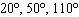3.

Draw a triangle with the given description.
a triangle with a 60° angle connected to an 80° angle by a 2-inch side

4.

Draw a quadrilateral with the given description.
a parallelogram with a 140° angle and a 5–centimeter side

5.

The design of a racing poster is shown.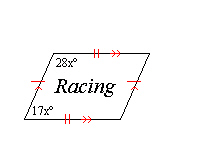a.   Identify the quadrilateral.
b.   Find the value of x.

Use a centimeter ruler to measure the segment shown. Find the scale of the drawing.

6.